Next: Charged Particle in a Up: Magnetism Previous: Ampère's Law

## The Lorentz Force

The flow of an electric current down a conducting wire is ultimately due to the movement of electrically charged particles (in most cases, electrons) along the wire. It seems reasonable, therefore, that the force exerted on the wire when it is placed in a magnetic field is simply the resultant of the forces exerted on these moving charges. Let us suppose that this is the case.

Letbe the (uniform) cross-sectional area of the wire, and let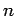be the number density of mobile charges in the wire. Suppose that the mobile charges each have charge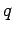and drift velocity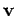. We must assume that the wire also contains stationary charges, of charge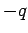and number density, say, so that the net charge density in the wire is zero. In most conductors, the mobile charges are electrons, and the stationary charges are atoms. The magnitude of the electric current flowing through the wire is simply the number of coulombs per second which flow past a given point. In one second, a mobile charge moves a distance, so all of the charges contained in a cylinder of cross-sectional areaand lengthflow past a given point. Thus, the magnitude of the current is. The direction of the current is the same as the direction of motion of the charges (i.e.,), so the vector current is. According to Eq. (152), the force per unit length acting on the wire is(157)

However, a unit length of the wire contains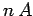moving charges. So, assuming that each charge is subject to an equal force from the magnetic field (we have no reason to suppose otherwise), the magnetic force acting on an individual charge is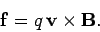(158)

This formula implies that the magnitude of the magnetic force exerted on a moving charged particle is the product of the particle's charge, its velocity, the magnetic field-strength, and the sine of the angle subtended between the particle's direction of motion and the direction of the magnetic field. The force is directed at right-angles to both the magnetic field and the instantaneous direction of motion.

We can combine the above equation with Eq. (65) to give the force acting on a chargemoving with velocityin an electric fieldand a magnetic field: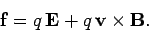(159)

This is called the Lorentz force law, after the Dutch physicist Hendrick Antoon Lorentz, who first formulated it. The electric force on a charged particle is parallel to the local electric field. The magnetic force, however, is perpendicular to both the local magnetic field and the particle's direction of motion. No magnetic force is exerted on a stationary charged particle.

The equation of motion of a free particle of chargeand massmoving in electric and magnetic fields is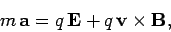(160)

according to the Lorentz force law. Here,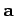is the particle's acceleration. This equation of motion was verified in a famous experiment carried out by the Cambridge physicist J.J. Thompson in 1897. Thompson was investigating cathode rays, a then mysterious form of radiation emitted by a heated metal element held at a large negative voltage (i.e., a cathode) with respect to another metal element (i.e., an anode) in an evacuated tube. German physicists maintained that cathode rays were a form of electromagnetic radiation, whereas British and French physicists suspected that they were, in reality, a stream of charged particles. Thompson was able to demonstrate that the latter view was correct. In Thompson's experiment, the cathode rays pass though a region of crossed electric and magnetic fields (still in vacuum). The fields are perpendicular to the original trajectory of the rays, and are also mutually perpendicular.Let us analyze Thompson's experiment. Suppose that the rays are originally traveling in the-direction, and are subject to a uniform electric fieldin the-direction, and a uniform magnetic fieldin the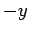-direction--see Fig. 23. Let us assume, as Thompson did, that cathode rays are a stream of particles of massand charge. The equation of motion of the particles in the-direction is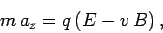(161)

whereis the velocity of the particles in the-direction, and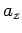the acceleration of the particles in the-direction. Thompson started off his experiment by only turning on the electric field in his apparatus, and measuring the deflection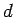of the rays in the-direction after they had traveled a distance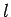through the field. Now, a particle subject to a constant accelerationin the-direction is deflected a distance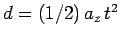in a time. Thus,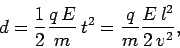(162)

where the time of flightis replaced by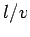. This replacement is only valid if(i.e., if the deflection of the rays is small compared to the distance they travel through the electric field), which is assumed to be the case. Next, Thompson turned on the magnetic field in his apparatus, and adjusted it so that the cathode rays were no longer deflected. The lack of deflection implies that the net force on the particles in the-direction is zero. In other words, the electric and magnetic forces balance exactly. It follows from Eq. (161) that, with a properly adjusted magnetic field-strength,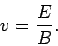(163)

Thus, Eqs. (162) and (163) can be combined and rearranged to give the charge to mass ratio of the particles in terms of measured quantities: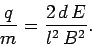(164)

Using this method, Thompson inferred that cathode rays are made up of negatively charged particles (the sign of the charge is obvious from the direction of the deflection in the electric field) with a charge to mass ratio of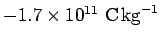. A decade later, in 1908, the American Robert Millikan performed his famous oil drop experiment in which he discovered that mobile electric charges are quantized in units ofC. Assuming that mobile electric charges and the particles which make up cathode rays are one and the same thing, Thompson's and Millikan's experiments imply that the mass of these particles iskg. Of course, this is the mass of an electron (the modern value iskg), andC is the charge of an electron. Thus, cathode rays are, in fact, streams of electrons which are emitted from a heated cathode, and then accelerated because of the large voltage difference between the cathode and anode.

If a particle is subject to a force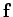which causes it to displace bythen the work done on the particle by the force is(165)

whereis the angle subtended between the force and the displacement. However, this angle is always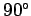for the force exerted by a magnetic field on a charged particle, since the magnetic force is always perpendicular to the particle's instantaneous direction of motion. It follows that a magnetic field is unable to do work on a charged particle. In other words, a charged particle can never gain or lose energy due to interaction with a magnetic field. On the other hand, a charged particle can certainly gain or lose energy due to interaction with an electric field. Thus, magnetic fields are often used in particle accelerators to guide charged particle motion (e.g., in a circle), but the actual acceleration is always performed by electric fields.Next: Charged Particle in a Up: Magnetism Previous: Ampère's Law
Richard Fitzpatrick 2007-07-14